## Sphere Packing

Letdenote the Packing Density, which is the fraction of a Volume filled by identical packed Spheres. In 2-D (Circle Packing), there are two periodic packings for identical Circles: square lattice and hexagonal lattice. Fejes Tóth (1940) proved that the hexagonal lattice is indeed the densest of all possible plane packings (Conway and Sloane 1993, pp. 8-9).

In 3-D, there are three periodic packings for identical spheres: cubic lattice, face-centered cubic lattice, and hexagonal lattice. It was hypothesized by Keplerin 1611 that close packing (cubic or hexagonal) is the densest possible (has the greatest), and this assertion is known as the Kepler Conjecture. The problem of finding the densest packing of spheres (not necessarily periodic) is therefore known as the Kepler Problem. The Kepler Conjecture is intuitively obvious, but the proof remained elusive until it was accomplished in a series of papers by Hales culminating in 1998. Gauß(1831) proved that the face-centered cubic is the densest lattice packing in 3-D (Conway and Sloane 1993, p. 9). This result has since been extended to Hypersphere Packing.

In 3-D, face-centered cubic close packing and hexagonal close packing (which is distinct from hexagonal lattice), both give(1)

For packings in 3-D, C. A. Rogers (1958) showed that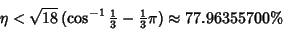(2)

(Le Lionnais 1983). This was subsequently improved to 77.844% (Lindsey 1986), then 77.836% (Muder 1988). However, Rogers (1958) remarks that many mathematicians believe, and all physicists know'' that the actual answer is 74.05% (Conway and Sloane 1993, p. 3).

Random'' close packing in 3-D gives only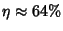(Jaeger and Nagel 1992).

The Packing Densities for several packing types are summarized in the following table.

 Packing(exact)(approx.) square lattice (2-D)0.7854 hexagonal lattice (2-D)0.9069 cubic lattice0.5236 hexagonal lattice0.6046 face-centered cubic lattice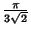0.7405 random -- 0.6400For cubic close packing, pack six Spheres together in the shape of an Equilateral Triangle and place another Sphere on top to create a Triangular Pyramid. Now create another such grouping of seven Spheres and place the two Pyramids together facing in opposite directions. A Cube emerges. Consider a face of the Cube, illustrated below.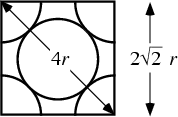The unit cell'' cube contains eight-spheres (one at each Vertex) and six Hemispheres. The total Volume of Spheres in the unit cell is(3)

The diagonal of the face is, so each side is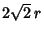. The Volume of the unit cell is therefore(4)

The Packing Density is therefore(5)

(Conway and Sloane 1993, p. 2).

Hexagonal close packing must give the same values, since sliding one sheet of Spheres cannot affect the volume they occupy. To verify this, construct a 3-D diagram containing a hexagonal unit cell with three layers. Both the top and the bottom contain six-Spheres and one Hemisphere. The total number of spheres in these two rows is therefore(6)

The Volume of Spheres in the middle row cannot be simply computed using geometry. However, symmetry requires that the piece of the Sphere which is cut off is exactly balanced by an extra piece on the other side. There are therefore three Spheres in the middle layer, for a total of six, and a total Volume(7)

The base of the Hexagon is made up of 6 Equilateral Triangles with side lengths. The unit cell base Area is therefore(8)

The height is the same as that of two Tetrahedra lengthon a side, so(9)

giving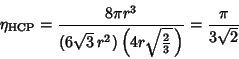(10)

(Conway and Sloane 1993, pp. 7 and 9).

If we had actually wanted to compute the Volume of Sphere inside and outside the Hexagonal Prism, we could use the Spherical Cap equation to obtain(11)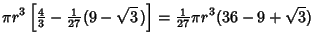(12)

The rigid packing with lowest density known has(Gardner 1966). To be Rigid, each Sphere must touch at least four others, and the four contact points cannot be in a single Hemisphere or all on one equator.

If spheres packed in a cubic lattice, face-centered cubic lattice, and hexagonal lattice are allowed to expand, they form cubes, hexagonal prisms, and rhombic dodecahedra. Compressing a random packing gives polyhedra with an average of 13.3 faces (Coxeter 1958, 1961).

For sphere packing inside a Cube, see Goldberg (1971) and Schaer (1966).

See also Cannonball Problem, Circle Packing, Dodecahedral Conjecture, Hemisphere, Hermite Constants, Hypersphere, Hypersphere Packing, Kepler Conjecture, Kepler Problem, Kissing Number, Local Density, Local Density Conjecture, Sphere

References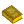Sphere Packings

Conway, J. H. and Sloane, N. J. A. Sphere Packings, Lattices, and Groups, 2nd ed. New York: Springer-Verlag, 1993.

Coxeter, H. S. M. Close-Packing and so Forth.'' Illinois J. Math. 2, 746-758, 1958.

Coxeter, H. S. M. Close Packing of Equal Spheres.'' Section 22.4 in Introduction to Geometry, 2nd ed. New York: Wiley, pp. 405-411, 1961.

Coxeter, H. S. M. The Problem of Packing a Number of Equal Nonoverlapping Circles on a Sphere.'' Trans. New York Acad. Sci. 24, 320-331, 1962.

Critchlow, K. Order in Space: A Design Source Book. New York: Viking Press, 1970.

Cundy, H. and Rollett, A. Mathematical Models, 3rd ed. Stradbroke, England: Tarquin Pub., pp. 195-197, 1989.

Eppstein, D. Covering and Packing.'' http://www.ics.uci.edu/~eppstein/junkyard/cover.html.

Fejes Tóth, G. Über einen geometrischen Satz.'' Math. Z. 46, 78-83, 1940.

Fejes Tóth, G. Lagerungen in der Ebene, auf der Kugel und in Raum, 2nd ed. Berlin: Springer-Verlag, 1972.

Gardner, M. Packing Spheres.'' Ch. 7 in Martin Gardner's New Mathematical Diversions from Scientific American. New York: Simon and Schuster, 1966.

Gauss, C. F. Besprechung des Buchs von L. A. Seeber: Intersuchungen über die Eigenschaften der positiven ternären quadratischen Formen usw.'' Göttingsche Gelehrte Anzeigen (1831, July 9) 2, 188-196, 1876.

Goldberg, M. On the Densest Packing of Equal Spheres in a Cube.'' Math. Mag. 44, 199-208, 1971.

Hales, T. C. The Sphere Packing Problem.'' J. Comput. Appl. Math 44, 41-76, 1992.

Jaeger, H. M. and Nagel, S. R. Physics of Granular States.'' Science 255, 1524, 1992.

Le Lionnais, F. Les nombres remarquables. Paris: Hermann, p. 31, 1983.

Lindsey, J. H. II. Sphere Packing in.'' Math. 33, 137-147, 1986.

Muder, D. J. Putting the Best Face of a Voronoi Polyhedron.'' Proc. London Math. Soc. 56, 329-348, 1988.

Rogers, C. A. The Packing of Equal Spheres.'' Proc. London Math. Soc. 8, 609-620, 1958.

Rogers, C. A. Packing and Covering. Cambridge, England: Cambridge University Press, 1964.

Schaer, J. On the Densest Packing of Spheres in a Cube.'' Can. Math. Bul. 9, 265-270, 1966.

Sloane, N. J. A. The Packing of Spheres.'' Sci. Amer. 250, 116-125, 1984.

Stewart, I. The Problems of Mathematics, 2nd ed. Oxford, England: Oxford University Press, pp. 69-82, 1987.

Thompson, T. M. From Error-Correcting Codes Through Sphere Packings to Simple Groups. Washington, DC: Math. Assoc. Amer., 1984.